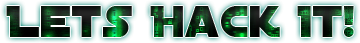# Decoding Frame Data

Now we’re ready to decode the frame data from the Convolution Code. For that we will use an algorithm called Viterbi. Viterbi is an algorithm to find hidden values in markov sequences. The Trellis Diagram from a Convolution Code is basically a markov sequence with hidden state (the hidden state is our data). Luckly the libfec includes a viterby for convolution encoded code with k=7 and rate=1/2. We’re going to use it. Its pretty straightforward to use it, but we need to have the frames in sync and know the frame period. You can discover the frame period (if you’re already didn’t) by checking the distance between sync words). In case of LRIT the frames are 8192 bits wide (1024 bytes) from which 32 bits (4 bytes) is the sync word. Since its convolution encoded with rate 1/2, we will have a encoded frame size of 16384 bits (or 2048 bytes). Using libfec we can decode it like this:

``````#include <fec.h>

#define VITPOLYA 0x4F
#define VITPOLYB 0x6D

uint8_t codedData; // Our coded frame data as soft symbols
uint8_t decodedData; // Our decoded frame
int viterbiPolynomial = {VITPOLYA, VITPOLYB};

/* */
void *viterbi;
set_viterbi27_polynomial(viterbiPolynomial);
if((viterbi = create_viterbi27(16384)) == NULL){
printf("create_viterbi27 failed\n");
exit(1);
}
// Now for each frame:
init_viterbi27(viterbi, 0);
update_viterbi27_blk(viterbi, codedData, 16384 + 6);
chainback_viterbi27(viterbi, decodedData, 16384, 0);
// decodedData will have the decoded stuff
``````

So as a side note now: Do you remember in the last chapter that I said about saving only the soft symbols? We synced using the soft symbols and viterbi will also use the soft symbols. What is the advantage? Since both Correlation Search and Viterbi are statistical algorithms, this increase the odds that a value that is on the middle of the decision tree (for example something close to 0 on a signed byte, that can be either bit 1 or bit 0) will not count much for the statistics. So a number that is on the middle can be either 0 or 1, viterbi will use that as a *I can be any value* in the trellis diagram and see which one is the most probably using other values in the sequence. This increases the quality of decoding the same as if you signal as about 2dB Higher SNR, and believe, that’s a HUGE improvement.

Usually after decoding a frame, you might also want to check the quality of the decoding. So a good way to measure is by re-encoding the signal and comparing the two streams of the original encoded signal and the expected encoded signal (the one you encoded).

``````/**/

void convEncode(uint8_t *data, int dataLength, uint8_t *output, int outputLen) {
unsigned int encstate = 0;
uint8_t c;
uint32_t pos = 0;
uint32_t opos = 0;

memset(output, 0x00, outputLen);
while (pos < dataLength && (pos * 16) < outputLen) {
c = data[pos];
for(int i=7;i>=0;i--){
encstate = (encstate << 1) | ((c >> 7) & 1);
c <<= 1;
output[opos]   = ~(0 - parity(encstate & viterbiPolynomial));
output[opos+1] = ~(0 - parity(encstate & viterbiPolynomial));

opos += 2;
}
pos++;
}
}

uint32_t calculateError(uint8_t *original, uint8_t *corrected, int length) {
uint32_t errors = 0;
for (int i=0; i<length; i++) {
errors += hardCorrelate(original[i], ~corrected[i]);
}

return errors;
}
/**/

convEncode(decodedData, 1024, correctedData, 16384);
uint32_t errors = calculateError(codedData, correctedData, 16384) / 2;
float signalErrors = (100.f * errors) / 8192;
/**/
``````

Then in the variable signalErrors you will have the percent of wrong bits that had been corrected in your frame output. After that if you output to a file and check with BitDisplay you will see something like this:The bitdisplay actually shows the 0 bits as white and 1 bits as black. So the overall perception is inverted. You can see the sync word pattern and just after that a pattern that looks like a counter. This counter is actually the frame counter. After that you can see a wide black bar and some small patterns on the right side of this bar. This is actually the 11 bit Virtual Channel ID. Since all channel IDs are usually lower than 64, most of the bits are 0.

Now if you strip the first 4 bytes of each 1024 byte frame, you will have a virtual channel frame from the satellite! In the next part I will show how to parse this frame data and extract the packets that will in the last chapter form our files. You can check my frame decoder code here:

https://github.com/racerxdl/open-satellite-project/blob/225a36d4144c0fe0704eb50a8fbc428914f654c0/GOES/network/decoder_tcp.c